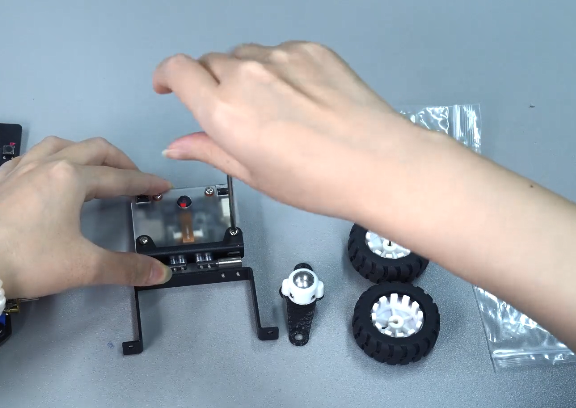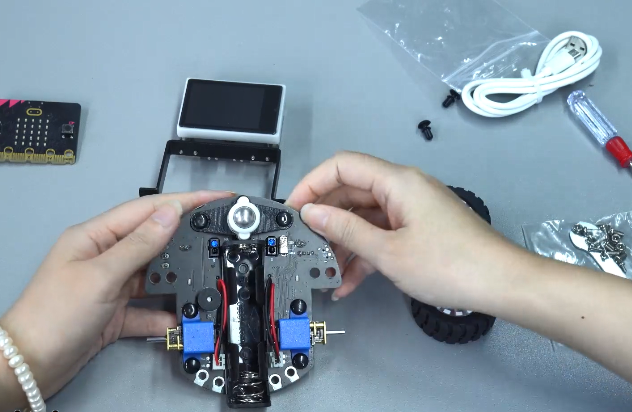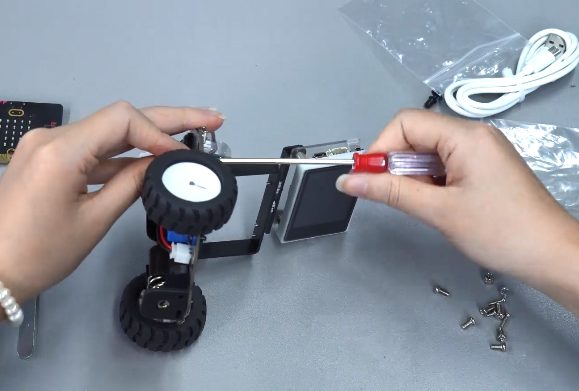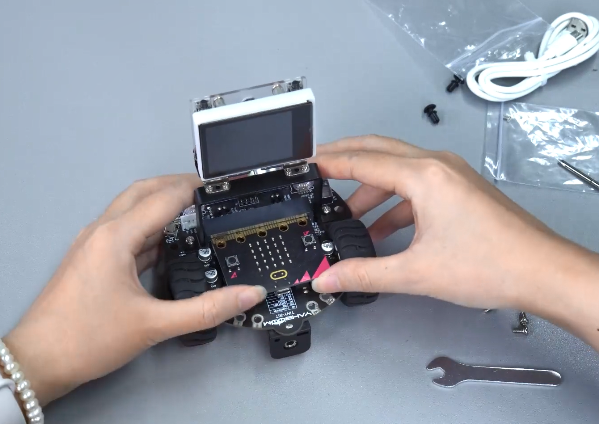### Yahboom Tiny:bit Pro micro:bit AI Vision Robot Car Platform

I tried using a robotic car "Tiny:bit Pro" that extends micro:bit provided by Yahboom.

The packing list includes: packing box, instruction manual, car extension board(with motor and mounting bracket), tires, infrared remote controller, rechargeable battery, tracking map, TF card+card reader.! ! !Note: If you purchase with Microbit board, your package will include Micro:bit board. If you purchase not include Micro:bit, you need prepare BBC Micro:bit by yourself.

Tiny:bit Pro have two option: Standard Kit / Expansion Kit

### Assembly

1.Remove the M3*6mm screws on the K210 module. And install the M3*6mm screw fixing bracket to the K210 module.2. Install universal wheel3. Install Trie4. Install K210 module on Tinybit car5. Insert Microbit boardCompletion Something cute and fuuny! Do you like this "guy"?

### SpecificationFront: Ultrasonic, sound sensor, high-brightness searchlight, infrared remote control receiver, programmable colorful RGB lights, alligator clip interface, PH2.0-4PIN serial port interface.

Back: Tracking sensor, buzzer, motor socket, serial port switch.

### Addition of Tiny:bit Pro block for MakeCode

Since it is a robot car that extended Micro:bit, it can block coding with MakeCode.

How to add Tiny:bit Pro blockVarious basic sample codes for Tinybit Pro are uploaded below.

RGB-light-exchange-lighting: https://makecode.microbit.org/_EL3LmqJTwKKT

Car-move-control-speed: https://makecode.microbit.org/_9oJaEzErWisY

Ultrasonic-avoid-with-light-sound: https://makecode.microbit.org/_P7WYcMXvUTqt

Voice control light: https://makecode.microbit.org/_KDDPHmJcaRut

Spinning top: https://makecode.microbit.org/_Wv0dPq5K8bFV

Various AI vidsion sample codes for Tinybit Pro are uploaded below.

Color recognition-Microbit car code: https://makecode.microbit.org/_1p84eJ9VxUkK

Color recognition-K210 module code:

import sensor, image, time, lcd

from modules import ybserial
import time

serial = ybserial()
lcd.init()
sensor.reset()
sensor.set_pixformat(sensor.RGB565)
sensor.set_framesize(sensor.QVGA)
sensor.skip_frames(time = 100)
sensor.set_auto_gain(False)
sensor.set_auto_whitebal(False)
clock = time.clock()

print("Hold the object you want to track in front of the camera in the box.")
print("MAKE SURE THE COLOR OF THE OBJECT YOU WANT TO TRACK IS FULLY ENCLOSED BY THE BOX!")

# Capture the color thresholds for whatever was in the center of the image.
# 50x50 center of QVGA.
r = [(320//2)-(50//2), (240//2)-(50//2), 50, 50]
for i in range(50):
img = sensor.snapshot()
img.draw_rectangle(r)
lcd.display(img)

print("Learning thresholds...")
threshold = [50, 50, 0, 0, 0, 0] # Middle L, A, B values.
for i in range(50):
img = sensor.snapshot()
hist = img.get_histogram(roi=r)
lo = hist.get_percentile(0.01) # Get the CDF of the histogram at the 1% range (ADJUST AS NECESSARY)!
hi = hist.get_percentile(0.99) # Get the CDF of the histogram at the 99% range (ADJUST AS NECESSARY)!
# Average in percentile values.
threshold = (threshold + lo.l_value()) // 2
threshold = (threshold + hi.l_value()) // 2
threshold = (threshold + lo.a_value()) // 2
threshold = (threshold + hi.a_value()) // 2
threshold = (threshold + lo.b_value()) // 2
threshold = (threshold + hi.b_value()) // 2
for blob in img.find_blobs([threshold], pixels_threshold=100, area_threshold=100, merge=True, margin=10):
img.draw_rectangle(blob.rect())
img.draw_cross(blob.cx(), blob.cy())
img.draw_rectangle(r, color=(0,255,0))
lcd.display(img)

print("Thresholds learned...")
print("Start Color Recognition...")
while(True):
clock.tick()
img = sensor.snapshot()
for blob in img.find_blobs([threshold], pixels_threshold=100, area_threshold=100, merge=True, margin=10):
img.draw_rectangle(blob.rect())
img.draw_cross(blob.cx(), blob.cy())
x = str(blob.x())
y = str(blob.y())
w = str(blob.w())
h = str(blob.h())
print(threshold)
if len(x)<3 :
i_flag = 3-len(x)
x = "0"*i_flag + x
if len(y)<3 :
i_flag = 3-len(y)
y = "0"*i_flag + y
if len(w)<3 :
i_flag = 3-len(w)
w = "0"*i_flag + w
if len(h)<3 :
i_flag = 3-len(h)
h = "0"*i_flag + h

send_data ="\$"+"01"+ x+','+ y+','+ w+','+ h+','+"#"
#print(send_data)
for i in range(1,5):
serial.send(send_data)

time.sleep_ms(5)
lcd.display(img)
#print(clock.fps())

Handwritten_digital_control-Microbit car codehttps://makecode.microbit.org/_7e82r50rpfzC

Handwritten_digital_control-K210 module code:

import sensor, image, time, lcd
from maix import KPU
import gc

from modules import ybserial
import time
serial = ybserial()

lcd.init()
sensor.reset()
sensor.set_pixformat(sensor.RGB565)
sensor.set_framesize(sensor.QVGA)
sensor.set_windowing((224, 224))
sensor.skip_frames(time = 100)
clock = time.clock()

kpu = KPU()

msg_ =""
while True:
gc.collect()
img = sensor.snapshot()
img_mnist1=img.to_grayscale(1)
img_mnist2=img_mnist1.resize(112,112)
img_mnist2.invert()
img_mnist2.strech_char(1)
img_mnist2.pix_to_ai()

out = kpu.run_with_output(img_mnist2, getlist=True)
max_mnist = max(out)
index_mnist = out.index(max_mnist)
msg_ = str(index_mnist)
send_data ="\$"+"11"+ msg_ +','+"#"
time.sleep_ms(5)
serial.send(send_data)
score = KPU.sigmoid(max_mnist)
if index_mnist == 1:
if score > 0.999:
display_str = "num: %d" % index_mnist
print(display_str, score)
img.draw_string(4,3,display_str,color=(0,0,0),scale=2)
elif index_mnist == 5:
if score > 0.999:
display_str = "num: %d" % index_mnist
print(display_str, score)
img.draw_string(4,3,display_str,color=(0,0,0),scale=2)
else:
display_str = "num: %d" % index_mnist
print(display_str, score)
img.draw_string(4,3,display_str,color=(0,0,0),scale=2)

lcd.display(img)
kpu.deinit()

Case effect show

Reviews